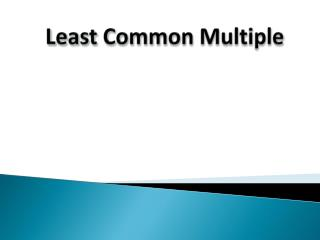# Least Common Multiple - PowerPoint PPT PresentationDownload PresentationLeast Common Multiple

Least Common MultipleDownload Presentation## Least Common Multiple

- - - - - - - - - - - - - - - - - - - - - - - - - - - E N D - - - - - - - - - - - - - - - - - - - - - - - - - - -
##### Presentation Transcript

1. Least Common Multiple

2. Least Common Multiple Definition: Least Common Multiple (LCM) – Is the smallest multiple that two or more numbershave in common.

3. Example 1: Find the LCM of 5 and 7 by listing the multiples. Multiples of 5: 5, 10, 15, 20, 25, 30, 35 Multiples of 7: 7, 14, 21, 28, 35, 42 35 is the least common multiple of 5 and 7 because it is the smallest number that both numbers go into. Least Common Multiple Method 1: Finding The LCM by Listing the Multiples Strategy: 1.) List the multiples of each number. 2.) Identify which smallest common multiple that both numbers have in common.

4. Least Common Multiple Method 2: Finding The LCM by Using a Table Strategy: 1.) Go through the prime numbers starting from 2. 2.) If a number is divisible by the prime number, then write the quotient. If it is not, leave the number alone. If you can use the number again, then do so. 3.) Work through the process until each number has a 1 in its column. 4.) Multiply the prime numbers in your table together to get the LCM. Example 2: Find the Least Common Multiple of 12 and 10. Prime Numbers 12 10 2 6 5 2 3 5 3 1 5 5 1 1 LCM: 2 x 2 x 3 x 5 = 60

5. Least Common Multiple Method 2: Finding The LCM by Using a Table Strategy: 1.) Go through the prime numbers starting from 2. 2.) If a number is divisible by the prime number, then write the quotient. If it is not, leave the number alone. If you can use the number again, then do so. 3.) Work through the process until each number has a 1 in its column. 4.) Multiply the prime numbers in your table together to get the LCM. Example 3: Find the Least Common Multiple of 4, 5 and 9. Prime Numbers 4 5 9 2 2 5 9 2 1 5 9 3 1 5 3 3 1 5 1 5 1 1 1 LCM: 2 x 2 x 3 x 3 x 5 = 180

6. Least Common Multiple Find the least common multiple of the following sets of numbers by using a table. 1.) 5 and 6 2.) 5, 9 and 12

7. Least Common Multiple Real-Life Application Mansi has painting class every two weeks. Riya has a pottery class every 5 weeks. Mansi and Riya met at the art building for class this week. How many weeks will it be until they see each other again at the art building?